# Riemann-Hurwitz formula

(Redirected from Riemann–Hurwitz formula)
Jump to: navigation, search

Hurwitz formula, Hurwitz theorem

A formula that connects the genus and other invariants in a covering of Riemann surfaces (cf. Riemann surface). Letandbe closed Riemann surfaces, and let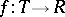be a surjective holomorphic mapping. Suppose this is an-sheeted covering, and suppose thatis branched in the points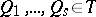with multiplicities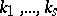. Suppose that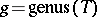and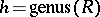. Then the following (Riemann–Hurwitz) formula holds:(*)

In particular, ifis the Riemann sphere, i.e.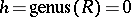, then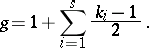Formula (*) was stated by B. Riemann  and proved by A. Hurwitz .

In the case of coverings of complete curves over a field, an analogous formula can be derived in case the covering mappingis separable (cf. Separable mapping). In that case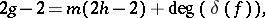whereis the different of. In this case one speaks of the Riemann–Hurwitz–Hasse formula. In case a branching multiplicity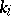is divisible by the characteristic of the base field, one speaks of wild ramification, and the degree ofat that point is larger than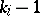.